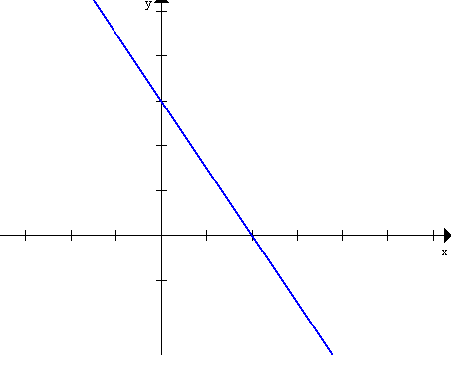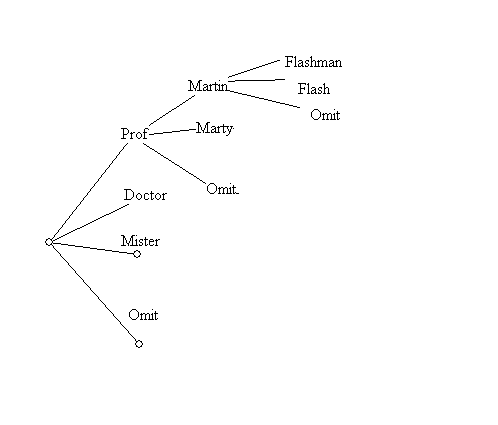Tuesday, September 26
First Class!

Unfortunately, I was not successful in transfering the notes I made during class. Hopefully I will do better in the future.

Here is my best recollection of most of what I had composed for notes.

• What is Visual Mathematics?
• Mathematics that studies topics related to visual experience.
• Visualization of mathematics that is not inherently visual.
• Example: Numbers... such as V, 5, five, cinq, chamesh, cinco
• Frege distinguished numerals from numbers in the late 19th century.
• We can compare numbers... for instance we say" 3 is less than 5"
• Is 3 smaller than 5?
• Numerals are symbols (visual or linguistic) that we use to represent numbers.
• We use numbers to measure (lengths) and put things in order (which was first).
• Another common visual representaion of numbers uses the number line.
• ___.___.___.___.___.___.___.______
•       1     2     3     4     5     6     7

• Here there numerals are connected to points, so the points are considered to visualize the corresponding numbers.

• We visualize equations that  give relations between numbers with graphs in the coordinate plane.

• 3x + 2y = 6 is visualized by the graph of a line ...• My Name: Martin Flashman
• How to start a letter to me:
•  Professor Martin Doctor Marty Flashman Mister Flash omit Omit Omit
• How many different openings are possible? We can visualize this problem with a "tree"
•• This visualization allows us to count the possibilities easily...

• seeing there are 8 possibilities for each of 4 title branches
so that the total is 8*4 = 32 possibilities.
• This is an example of a visualization used to understand and solve a problem that initially is not connected to anything visual .